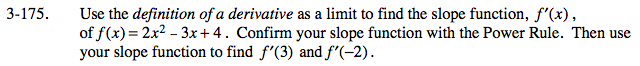### Home > CALC > Chapter Ch3 > Lesson 3.4.3 > Problem3-175

3-175.

Use the definition of a derivative as a limit to find the slope function, f ′(x), of f(x) = 2x2 − 3x + 4. Confirm your slope function with the Power Rule. Then use your slope function to find f ′(3) and f ′(−2). Homework Help ✎Hana's definition of the derivative:

$f'(x) =\lim_{h\rightarrow 0}\frac{f(x+h)-f(x)}{h}$

1. Substitute f(x) into Hana's definition
2. Expand the numerator
3. Combine like terms
4. Factor out an h
5. Cancel out the h
6. Evaluate the limit as h→0
This is f '(x)

Use the Power Rule to find f '(x). Compare to the f '(x) you found algebraically. If they do not agree, you probably made an algebraic error.

Evaluate f '(3) and f '(−3).